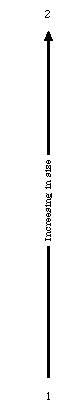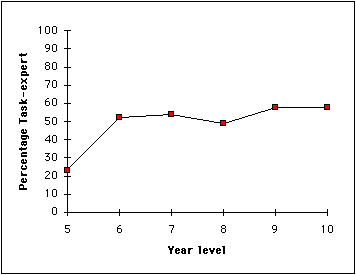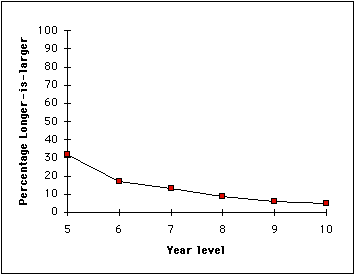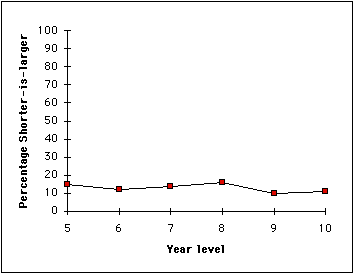Students' Understanding of Decimals: An Overview

### University of Melbourne

Although students first learn about decimal notation in primary schools, it is well known that secondary students in many countries, including Australia, do not have an adequate knowledge of the concepts involved. Even some students who can calculate with decimals do not understand the comparative size of the numbers involved.

Understanding decimal notation is an important part of basic numeracy. Our society makes widespread use of metric measurement for scientific and everyday purposes. Computers and calculators use decimal digital displays so making sense of input and output of decimal numbers is essential.

In this article, we will demonstrate some of the ways in which students think about decimal notation and how this changes as students get older. Our testing at one school, which seems to us to be quite a typical Australian high school, showed that about a quarter of students still had important misconceptions in Year 10. We present a simple test that teachers can use to help them diagnose the mistakes that their students are making and we discuss some of the help that is appropriate. In this article "decimals" will refer to "decimal fractions" and "fractions" will refer to "common fractions".

### Understanding decimals

Understanding decimals is a multidimensional task. Students need to co-ordinate place value concepts with aspects of whole number and fraction knowledge. Making the transition to understanding decimals relies on having a thorough understanding of previous concepts fully integrated with new information.

In this paper, we are concerned with whether students know the relative size of decimals well enough to order correctly sets of decimals. For example, can students place in increasing order the decimals 3.214, 3.09 and 3.8? Only a few students have trouble ordering decimals when the integer parts are different, so all the examples we use here will have the same integer part. Research studies in England, France, Israel and the United States (cited in the references to this article) have established that there are two main patterns of erroneous thinking: called the longer-is-larger rule and the shorter-is-larger rule. In all of the studies, including our Australian studies (Moloney, 1994; Moloney and Stacey, 1996, Stacey and Steinle, 1998, Steinle and Stacey, in press), significant proportions of students were seen to be consistently following these erroneous rules. Students construct these rules for themselves when they are trying to assimilate new knowledge about decimals with what they already know about whole numbers, place value and fractions.

Longer-is-larger rule. When asked to order decimals, some students systematically choose the number with most digits after the decimal point as the largest. These students are said to be following the longer-is-larger rule. They would say 4.125 is greater than 4.7. Usually, this is because the whole number 125 is larger than 7. The decimal point is recognised but only as a separator and the decimal portion is often read as a whole number e.g. "four point one hundred and twenty five", rather than the conventional "four point one two five". Many of these students have effectively constructed a number line such as the one shown on the left of Figure 1. Researchers have observed that longer-is-larger rule students often have a weak understanding of whole number place value so that even with whole numbers they simply reason that longer means bigger. An indication of how these students would order decimals is given by the "number line" on the left of Figure 1. In showing the "number lines" in Figure 1, we are not implying that students are sufficiently explicitly aware of all the logical consequences of their thinking that they could offer such a number line. We also know that students will often have other special knowledge (e.g. that 0.5 is a half) that cuts across the application of their rules for uncommon numbers.

Shorter-is-larger rule. Some students consistently choose the number with fewer decimal places as the larger. This is called the shorter-is-larger rule. These students would say that 2.3 is greater than 2.67. Some of them correctly reason that the 3 is the number of tenths while the 67 is the number of hundredths, and then wrongfully conclude that any number of tenths is larger than any number of hundredths. Alternatively, some may confuse 0.3 with 1/3 and 0.67 with 1/67, or even 0.3 with -3 and 0.67 with -67, giving the same result for a different reason. In effect, these students have constructed one of the number lines as shown on the right of Figure 1. Shorter-is-larger rule students usually recognise that digits after the decimal point relate to fractions but they do not connect the size of the parts and the number of parts. For example, when asked to write 3/4 in decimal form some shorter-is-larger rule students wrote 3.4, 0.3 or 0.34.

There are approximately ten sources of confusion which results in students choosing longer decimals as larger or shorter decimals as larger. There are also variations in the precise way that they do this, for example how they treat zeros. Sometimes they make decisions in special cases for other reasons. A thorough discussion of this is given by Stacey and Steinle (1998).2 . . 1.6792 . . 1.999 1.998 . . 1.101 1.100 1.99 1.98 . . 1.51 1.50 1.49 . . 1.12 1.11 1.10 1.9 1.8 1.7 1.6 1.5 1.4 1.3 1.2 1.1 1 2 1.9 1.8 1.7 1.6 1.5 1.4 1.3 1.2 1.1 1.99 1.98 . . 1.51 1.50 1.49 . . 1.02 1.01 1.999 1.998 . . . 1.002 1.001 . . 1.6792 . . 1 2 1.1 1.2 1.3 1.4 1.5 1.6 1.7 1.8 1.9 1.10 1.11 . . 1.49 1.50 1.51 . . 1.98 1.99 1.100 1.101 . . . 1.998 1.999 . . 1.6792 . . 1 Order of decimals for a whole number student Order of decimals for a fraction rule student (two possibilities)

Figure 1. Common students' ideas of the order of decimals between 1 and 2

### Are these errors common in Australia?

We have carried out several studies to gather data on the ideas about decimals held by Australian students. Firstly we tested two classes of secondary school students twice, a year apart and looked at how their ideas changed over the year. Secondly we have tested many classes of students from Year 5 to Year 10 and looked at how the numbers of students making each type of error varied. The first study was carried out in a middle-class Catholic school in Melbourne. The second study involved a wide range of schools.

We have used tests similar to the one shown in Figure 5 and also more complicated tests that pinpoint more exactly the thinking of the students. The test in Figure 5 allows us to determine whether the students are following one of the longer-is-larger rules, one of the shorter-is-larger rules or whether they seem to be an expert at the task. We know from interviews that some of these 'task experts' do not understand decimals thoroughly even though they can correctly follow a rule to order them.

### Changes in a year of schooling

We tested 26 Year 7 students and 24 Year 9 students in mid-1992 and again one year later, when they were in Years 8 and 10. One third (8) of the Year 7 students and one half (12) of the year 10 students were 'task experts'; they got nearly all of the questions correct. We found that 4 of the Year 7 students and 2 of the Year 10 students used the longer-is-larger rule. A third (9) of the Year 7 students and one quarter (6) of the Year 10 students used the shorter-is-larger rule.

The test was measuring change over time without any formal intervention other than normal teaching. It was very disappointing to see that there was only a small change in understanding of decimal notation during the course of a complete school year. Only six of the fifty students changed categories. Five moved into the task expert category - two from the shorter-is-larger category and three previously uncategorised. Thirteen of the fifteen students remained in the shorter-is-larger category. All six longer-is-larger students stayed in their category.

This study demonstrated that there is a significant problem with misconceptions about decimals. It is of great concern that half of the students at Year 10, and a larger percentage at other year levels, did not compare decimals correctly. Their teachers had previously been unaware of the extent of this problem. It is also very surprising that only 6 out of 50 students showed any movement between categories over a full year of schooling. This provides evidence that students were responding to the items in a way which reflected a stable thought pattern which teaching during the year did not alter.

### The Year 5 to Year 10 study

In a subsequent study, we tested 2517 students in Years 5 to 10. Line graphs illustrating changes in the percentage of students in the task expert, longer-is-larger and shorter-is-larger categories from Years 5 to 10 are given in Figures 2, 3 and 4. The percentage of students classified as task expert rises reasonably steadily, but was still only 58% by Year 10 (see Figure 2). A large proportion of the younger students used the longer-is-larger rule (see Figure 3) but this reduced in the secondary school. There was a slow decrease in the use of the shorter-is-larger rule but, as Figure 4 shows, it remained in higher year levels (11% in Year 10 in this study). This misconception in particular has the potential to remain with students into adulthood unless it is challenged.Figure 2. Percentage of task expert students by year level.Figure 3. Percentage of longer-is-larger students by year level.Figure 4. Percentage of shorter-is-larger students by year level.

### Testing students' understanding

The test in Figure 5 is a quick test which teachers can use to identify most students with misunderstandings about decimal notation. The answers that students in each category will give are shown in Figure 6. The test was originally adapted it from Resnick et al (1989) but it has now gone through several stages of development. Students who are thinking about decimals with the longer-is-larger rule will tend to get the first five questions wrong and the second five correct. Allocate a student to this category if at least four out of the five answers match this prediction. Shorter-is-larger rule students will probably be correct for the first group of five questions and incorrect for the next five. Again use a four out of five criterion for making the classification. Students classified as Apparent-experts will get at least four out of five correct in each group of five questions.

Within each of these three categories, further decisions may be made on how the student is thinking by examining the last three items. For example, the response to item 11 will distinguish between students who simply treat decimal numbers as 2 whole numbers separated by a dot and students who have a slightly more sophisticated understanding, so that they realise decimals with a zero in the tenths column are small. Similarly, item 12 will distinguish between Shorter-is-larger students who think of decimals as reciprocals or negatives and those who look only at the length, as contrasted in the number lines of Figure 1. Finally, item 13 will help to separate students who can interpret two decimal places quite well (perhaps by analogy with money) from those who are able to make correct decisions throughout the test (for whatever reason!).

For each pair of decimal numbers, circle the LARGER.

 4.8 4.63 0.5 0.36 0.75 0.8 0.37 0.216 3.92 3.4813 5.62 5.736 0.6 0.85 0.426 0.3 2.516 2.8325 7.942 7.63 4.08 4.7 1.85 1.84 17.353 17.35

Figure 5. Test of understanding of decimal notation

 Number pair Longer-is-larger misconceptions Shorter-is-larger misconceptions Apparent-expert behaviour 4.8 4.63 X C C 0.5 0.36 X C C 0.75 0.8 X C C 0.37 0.216 X C C 3.92 3.4813 X C C 5.62 5.736 C X C 0.6 0.85 C X C 0.426 0.3 C X C 2.516 2.8325 C X C 7.942 7.63 C X C 4.08 4.7 ? C C 1.85 1.84 C ? C 17.353 17.35 C X ?

Figure 6 Responses given by students in the major misconception categories. Correct (C), incorrect (X) and variable (?)

### Using the results

When students' misconceptions about decimals have been classified, teachers may be able to target their teaching to particular groups of students. From our results, it is clear that students are able to carry out the standard tasks of arithmetic at school without having their understanding of the fundamental meanings of decimal notation challenged. They can carry out routine procedures without developing a real sense of the size of the numbers and without indicating to their teachers the depth of their misconceptions. Students must be continually challenged as to the reasonableness of any answers obtained: "Does this answer make sense?"

It is important that students are not able to mask misunderstanding of decimals by carrying out routine procedures such as adding zeros to the end of decimals to compare them e.g. to compare 0.3 with 0.12, change 0.3 to 0.30 and then compare the whole numbers 30 and 12. We advocate doing plenty of calculations (by hand and by calculator) which use decimals of varying lengths in the one question. If students only operate on decimals with one decimal place, say for months, they need never learn how decimals with different numbers of decimal places compare.

Longer-is-larger students may need quite basic assistance with ideas of place value for whole number. Teachers will need to discuss the reasons for referring to a decimal such as 0.29 as "nought point two nine" rather than "nought point twenty nine". Counting (e.g. by 0.1) with the support of a calculator's constant addition facility can reinforce the idea that 0.9 is followed by 1.0 (and not 0.10, "nought point ten").

Positioning numbers on a number line is useful for students with either misconception. Facility with relationships based on place value are essential. For example, it is critical that students learn that 0.7 is equivalent to 0.70 and why it is so. Shorter-is-larger rule students especially need to learn to consider both the magnitude of the parts of a fraction (e.g. tenths for 0.4) and the number of parts (e.g. 4 for 0.4) to appreciate the size of a decimal. A variety of concrete aids (even colouring in squares on a 10x10 grid) is useful here. Converting from fractions to decimals and vice versa, by calculator or otherwise, can be useful, provided students carefully consider the answers rather than simply working them out!

The overall finding of these studies, that a very significant proportion of Year 10 students cannot reliably decide which of a pair of decimals is the larger, is disturbing. In our metric, calculator world an understanding of decimal notation is a high priority for all students.

### References

Moloney, K. (1994). The Evolution of Concepts of Decimals in Primary and Secondary Students. Unpublished M. Ed. thesis: University of Melbourne.

Moloney, K. & Stacey, K. (1996). Understanding Decimals. The Australian Mathematics Teacher, 52(1), 4-8.

Nesher, P. & Peled, I. (1986). Shifts in Reasoning. Educational Studies in Mathematics, 17, 67-79.

Resnick, L., Nesher, P., Leonard, F., Magone, M., Omanson S. & Peled, I. (1989). Conceptual Bases of Arithmetic Errors : The Case of Decimal Fractions. Journal for Research in Mathematics Education, 20(1), 8-27.

Sackur-Grisvard, C. & Leonard, F. (1985). Intermediate Cognitive Organizations in the Process of Learning a Mathematical Concept: The Order of Positive Decimal Numbers. Cognition and Instruction, 2(2), 157-174.

Stacey, K. & Steinle, V. (1998). Refining the Classification of Student's Interpretations of Decimal Notation. Hiroshima Journal of Mathematics Education, 6, 49-69.

Steinle, V. & Stacey, K. (in press) The incidence of misconceptions of decimal notation amongst students in Grades 5 to 10. In Proceedings of the 1998 Annual Conference of the Mathematics Education Research Group of Australia.

### Acknowledgement

The authors would like to thank the teachers and students who generously gave their time to participate in these studies.